Samacheer Kalvi 10th Maths Solutions Chapter 8 Statistics and Probability Ex 8.2

Tamilnadu Samacheer Kalvi 10th Maths Solutions Chapter 8 Statistics and Probability Ex 8.2

10th Maths Exercise 8.2 Samacheer Kalvi Question 1.
The standard deviation and coefficient of variation of a data are 1.2 and 25.6 respectively. Find the value of mean.
Solution:
Co-efficient of variation C.V. = $$\mathrm{C.V}=\frac{\sigma}{\overline{x}} \times 100$$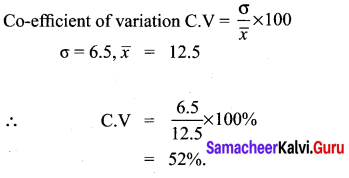10th Maths Exercise 8.2 Question 2.
The standard deviation and coefficient of variation of a data are 1.2 and 25.6 respectively. Find the value of mean.
Solution: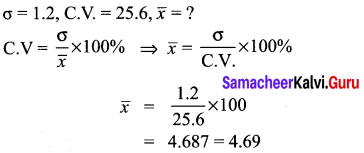Class 10th Math Exercise 8.2 Question 3.
If the mean and coefficient of variation of a data are 15 and 48 respectively, then find the value of standard deviation.
Solution: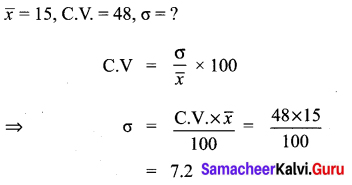Ex 8.2 Class 10 Question 4.
If n = 5 , $$\overline{x}$$ = 6, $$\Sigma x^{2}$$ = 765, then calculate the coefficient of variation.
Solution: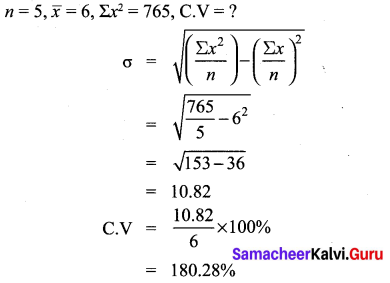8.2 Class 10 Question 5.
Find the coefficient of variation of 24, 26, 33, 37, 29,31.
Solution: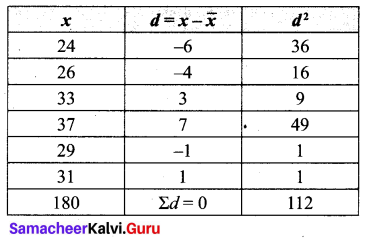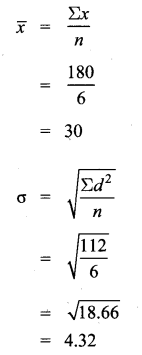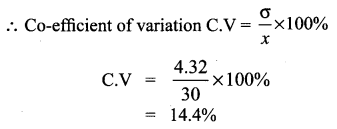Exercise 8.2 Class 10 Question 6.
The time taken (in minutes) to complete a homework by 8 students in a day are given by 38, 40, 47, 44, 46, 43, 49, 53. Find the coefficient of variation.
Solution: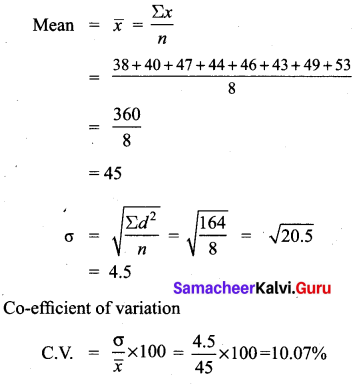10th Maths Statistics And Probability Question 7.
The total marks scored by two students Sathya and Vidhya in 5 subjects are 460 and 480 with standard deviation 4.6 and 2.4 respectively. Who is more consistent in performance?
Solution: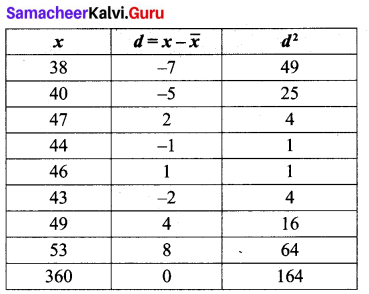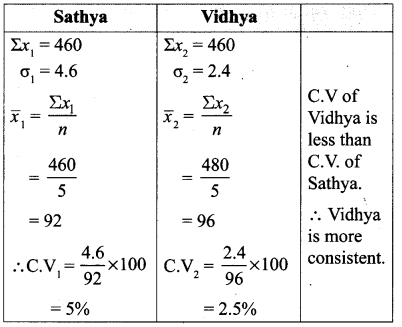10th Class Exercise 8.2 Question 8.
The mean and standard deviation of marks obtained by 40 students of a class in three subjects Mathematics, Science and Social Science are given below.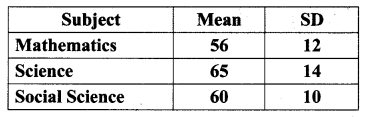Which of the three subjects shows highest variation and which shows lowest variation in marks?
Solution: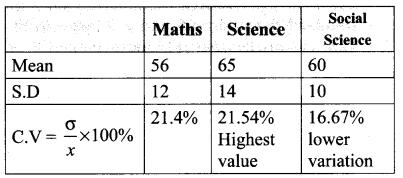Science subject shows highest variation. Social science shows lowest variation.

10th Math 8.2 Question 9.
The temperature of two cities A and B in a winter season are given below.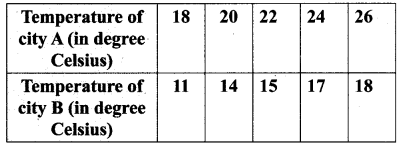Find which city is more consistent in temperature changes?
Solution: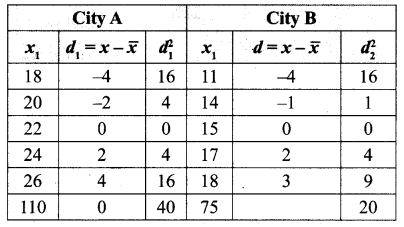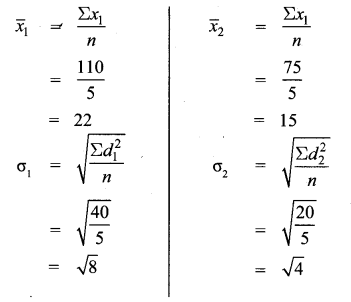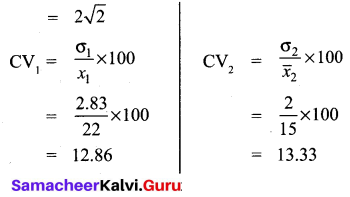∴ Co-efficient of variation of City A is less than C.V of City B.
∴ City A is more consistent.